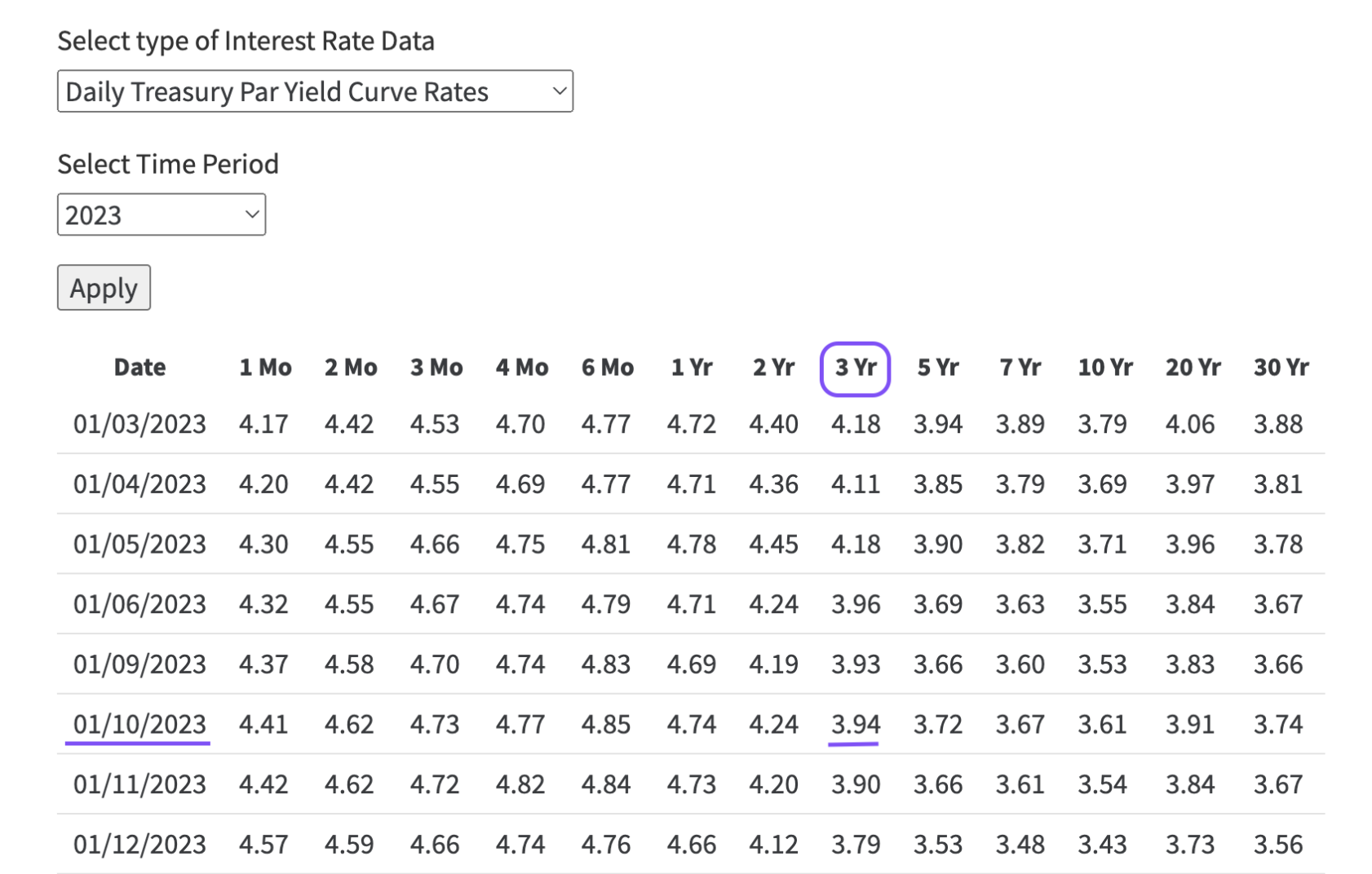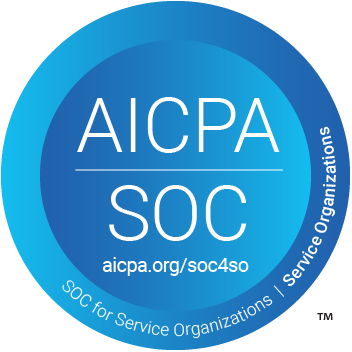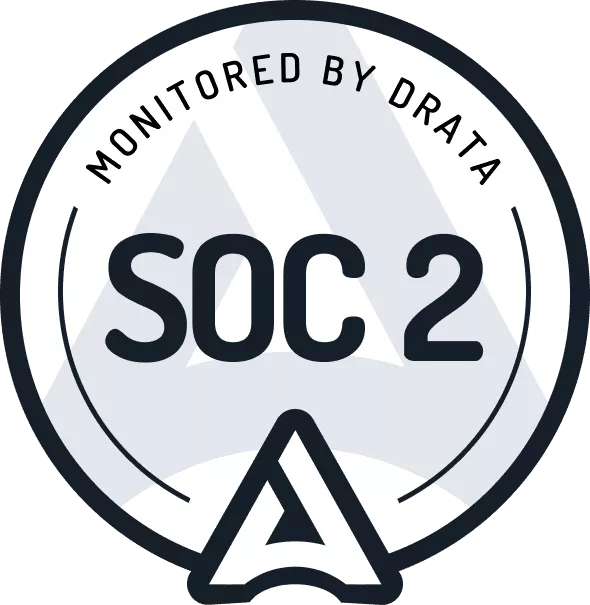# How to Calculate the Discount Rate Using the Risk Free Rate

by Lucas Russell | 2023-04-19

## What is the Risk Free Rate

This is what ASC 842 states when determining the discount rate:

842-20-30-3 A lessee should use the rate implicit in the lease whenever that rate is readily determinable. If the rate implicit in the lease is not readily determinable, a lessee uses its incremental borrowing rate. A lessee that is not a public business entity is permitted to use a risk-free discount rate for the lease, determined using a period comparable with that of the lease term, as an accounting policy election for all leases.

If you're looking for the most straight forward way to determine the discount rate in compliance with ASC 842 it's using the risk free rate.

## How to Calculate the Risk Free Rate

First, you can get your risk free rates here. Now that you have the risk free rates to determine the discount rate there are two inputs:

• Commencement/transition date of the lease
• Term of the lease

With these two inputs, you can now determine the risk free rate of the lease.

## Example

Let's say the lease commenced January 10, 2023 and the lease term is three years. The risk free rate is 3.94%.If the lease term does bond terms, use the average. For example for a 15 year lease, in this example you would use 3.76%.

## FooterHere at Cradle, our mission is simple; it's at the foundation of everything that we do. We want to make accountants' lives easier by leveraging technology to free up their time to focus on running the business.

140 Yonge St.
Suite 200
Toronto, ON M5C 1X6

US